• 검색 결과가 없습니다.

# Electrical Dipole Formation at Metal/Graphene Interface and Its Application for Junctionless CMOS

N/A
N/A
Protected

Share "Electrical Dipole Formation at Metal/Graphene Interface and Its Application for Junctionless CMOS "

Copied!
124
0
0
더 보기 ( 페이지)

전체 글

In true complementary MOS (CMOS) logic gate operation, the gate metal electrodes must be chosen to have effective work functions suitable for controlling both n-channel MOSFETs (NMOS) and p-channel MOSFETs (PMOS). Namely, the region boundary is formed at 𝑘𝑘𝑎𝑎=𝑛𝑛𝑛𝑛 with the integer value 𝑛𝑛 as shown in figure 2.1 which is the origin of the energy band gap of solid materials.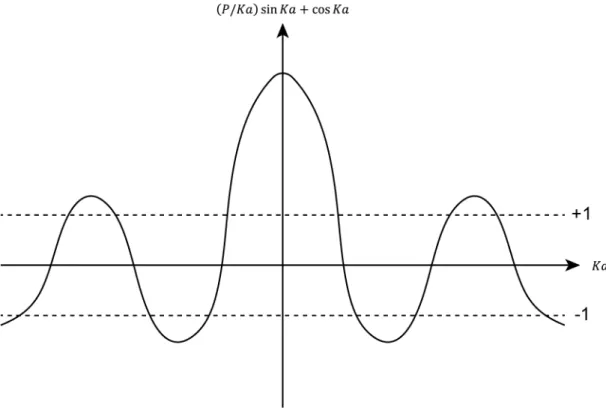Figure 2.1 Allow range of 𝐾𝐾𝑎𝑎 value calculated from the Kronig-Penny model.

## Metal/Semiconductor Junction

### Electrostatic Analysis for Energy Band Profile

Where 𝑄𝑄𝑠𝑠 is the surface charge density on the semiconductor due to depletion, 𝑊𝑊𝐷𝐷 the depletion width, 𝜖𝜖0 the vacuum permittivity, 𝜖𝜖𝑠𝑠 the dielectric constant of the semiconductor, 𝜙 𝜙𝐵𝐵 is the Schottky barrier height and 𝑉𝑉𝑛𝑛 is the energy difference. between 𝐸𝐸𝐶𝐶 and 𝐸𝐸𝐹𝐹 for n-type semiconductors. In this formalism, we can see that the main factor for determining 𝜙𝜙𝐵𝐵 is the work function of the metal 𝜙𝜙𝑚𝑚.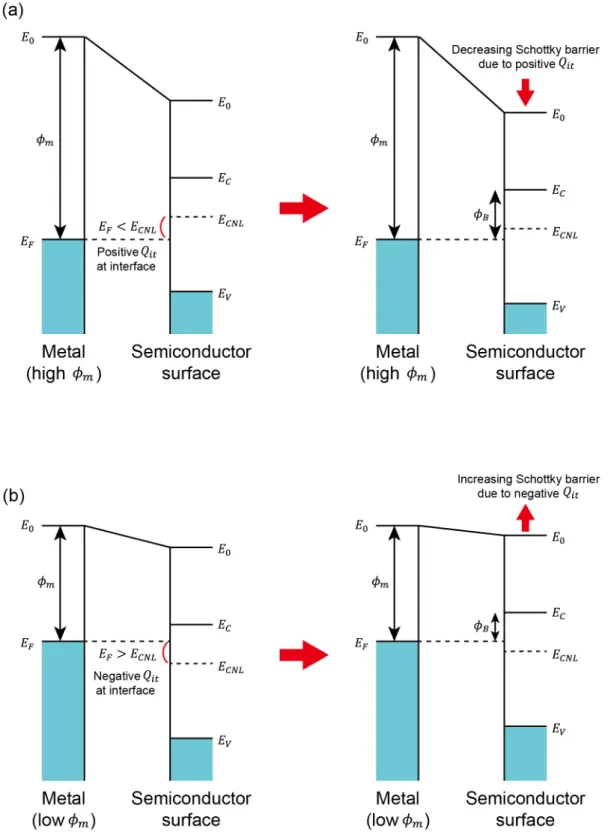Figure 2.5 Fermi-level pinning effect induced by the interface trap state for (a) the high work-function metal and (b) low work-function metal

### Current-Voltage Measurement

Where 𝐽𝐽𝑠𝑠→𝑚𝑚 is the current density flowing from the semiconductor to the metal, 𝐸𝐸𝐹𝐹+ in the 𝑥𝑥 direction. Therefore, we obtain the thermionic emission current density with the bias voltage by adding equation 2.52 and 2.53.

### Internal Photoemission Spectroscopy Measurement

If we use the metal electrode thin enough to allow most of the photons to penetrate the metal/semiconductor interface, it is reasonable to assume that 𝑘𝑘(ℎ𝜈𝜈) does not depend on the photon wavelength. Also, according to Powell's functional form , 𝑌𝑌(ℎ𝜈𝜈) from the metal electrode can be expressed as the square of the energy. Therefore, the Schottky barrier height 𝜙𝜙𝐵𝐵 can be derived from the linear fit of the 𝑌𝑌1/2 vs ℎ𝜈𝜈 plot.

## Metal/Oxide/Semiconductor Junction

### Electrostatic Analysis for Energy Band Profile

In a real MOS junction, there is a fixed oxide charge due to bulk traps or impurities that causes a voltage shift in the flat band. Therefore, we need further investigation of the band diagram with different bias voltage applied to the metal. Basically, we can define the surface potential Ψ as the electrostatic potential difference between the bulk and the surface of the semiconductor.

Ψ can be changed by applying a bias to the metal and the charge state of the semiconductor can be analyzed into multiple states with respect to the surface potential. Energy band diagrams with corresponding charge distributions versus DC bias are shown in Figure 2.9.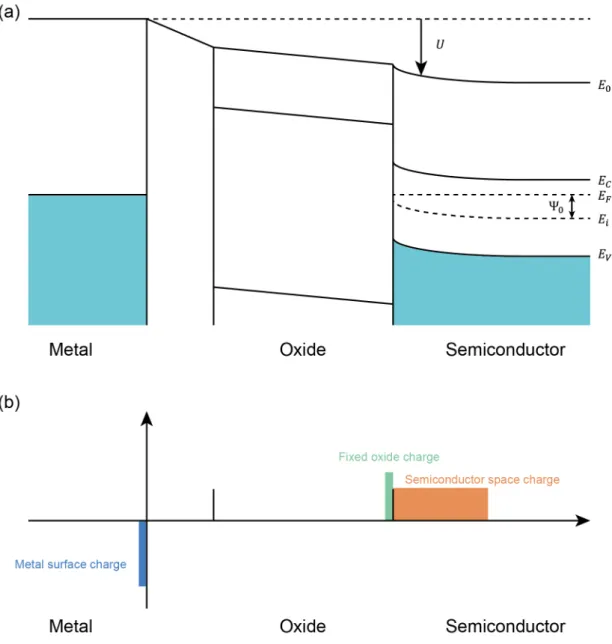Figure 2.8 (a) Band diagram across the MOS junction and (b) its surface charge distribution

Capacitance-Voltage Measurement

## Graphene

### Crystal and Energy Band Structure

Furthermore, the metal/graphene/semiconductor junction can be a suitable platform to observe the effective tuning of the metal work function induced by a graphene layer because the physics of direct carrier transport has been widely investigated with Schottky barrier formation. In this chapter, effective work function modulation with graphene interlayer is presented using numerical calculation and experimental analysis. The charge density of equation 3.1 represents the doping concentration of graphene due to free carrier transport.

If the metal forms a contact with the graphene, there is free carrier transfer due to their work function difference. When the metal with higher work function will induce the p-doped graphene if we only consider the free carrier transport.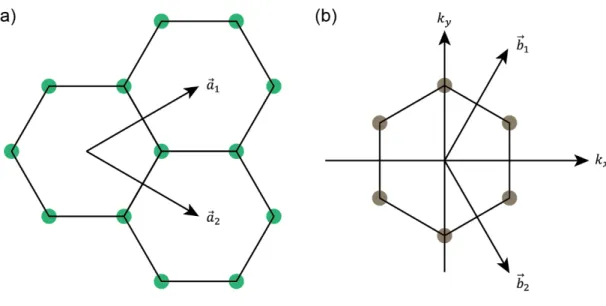Figure 2.11 (a) Lattice structure and (b) reciprocal lattice in the momentum space of graphene 

## Enhanced Fermi-level Pinning Effect Induced by Graphene Interlayer Calculated in

𝑉𝑉𝑛𝑛 is the energy difference between conduction band minimum and Fermi level of n-type Si at the bulk. The boundary conditions and the connection structures are illustrated in figure 3.2 and the material parameters used in the calculation are summarized in table 3.1. As a result, the Schottky barrier with the different metal work functions and number of graphene layer is calculated as shown in figure 3.3.

However, 𝑆𝑆 becomes almost zero when the four layers of graphene are inserted at the junction. This implies that the junction is strongly pinned due to the charge neutrality point of graphene multilayer.

## Asymmetric Orbital Overlap Dipole Layer Formation Between Metal and Graphene Interface

### Sample Fabrication

A conventionally n-doped GaAs substrate with a donor density of 5 × 1016 cm−3 was cleaned in a 1:1 NH4OH/H2O solution for one minute, then rinsed with DI water. To form the substrate ohmic contact, 5/20/500 nm thick Ti/Pt/Au was deposited in situ using e-beam evaporation and then annealed in vacuum at 400 °C for 5 min. After cleaning the substrate, monolayer graphene was transferred using a semi-dry transfer method reported in .

Then, the circular metal electrodes with a diameter of 500 µm and a thickness of 50 nm were deposited using e-beam evaporation. As a result, the metal/graphene/GaAs transition was formed in the region where graphene was transferred, and the metal/GaAs transition was formed in the other region in the same substrate, as shown in Figure 3.4.

### Measurement Results

From the numerical calculation performed in the metal/multilayer graphene/Si junction as discussed in Chapter 3.1, we expected that the Schottky barrier will be increased for low work function metal and reduced for high work function metal due to the free carrier transport with graphene. To find additional information about the intersections, we performed the IPE measurement with the configuration as shown in Figure 3.6 and the measured IPE data is shown in Figure 3.7. In the IPE measurement, the small reverse bias of 1 mV is applied to the GaAs substrate to make it easy for hot electrons to move into the GaAs substrates.

In the case of Al, the measured Schottky barrier is 1.004 eV, considering the effect of reducing the image power on the IPE threshold. Based on the measurement results, it is reasonable to assume that the GaAs surface has a local variation of the interstitial trap states because the large interstitial trap state can screen most of the charges in the metal and graphene.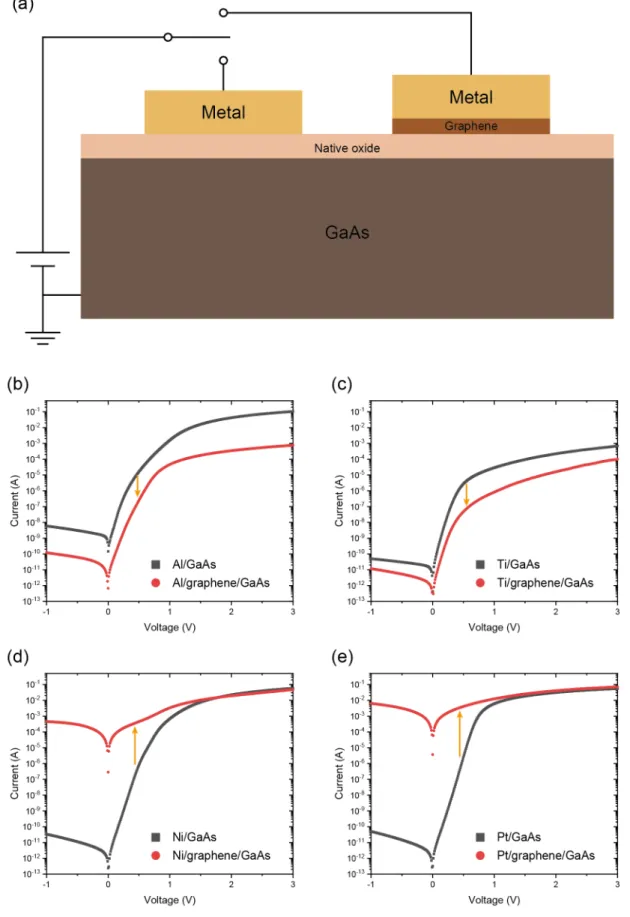Figure 3.5 (a) Configuration of I-V measurement and measured I-V curves for (b) Al, (c) Ti, (d) Ni and (e) Pt metal electrodes 

### Electrostatic Band Profile Analysis Using Finite Element Method

This is confirmed with the high-resolution transmission electron microscope (HRTEM) image as shown in Figure 3.13. And the quality of the transferred graphene was confirmed by the Raman spectroscopy, as shown in Figure 3.14. The surface charge distributions and the potential with the material parameters are shown in Figure 3.16.

The measurement configuration and the measured R-T curves with the different thicknesses are shown in figure B.3. As shown in figure B.3. all movies show the disordered metal behavior with the negative slope.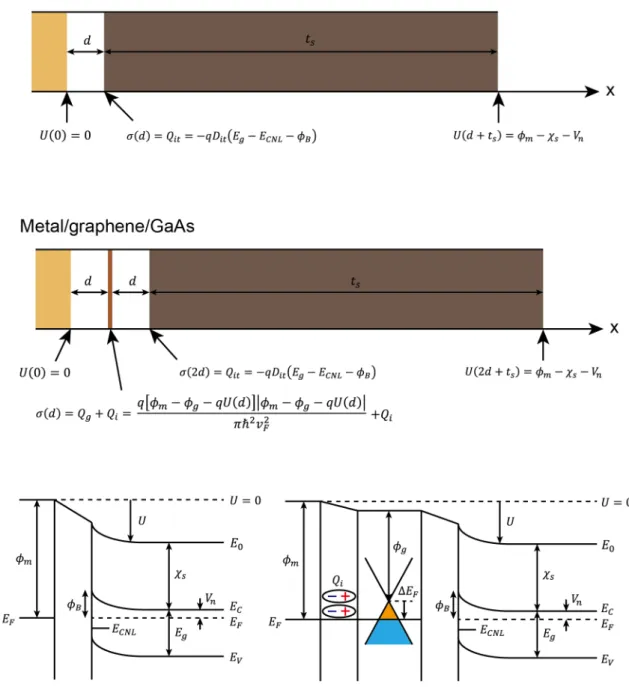Figure 3.9 Boundary conditions and definition of variables for the metal/GaAs and metal/graphene/GaAs junctions assumed in the finite element calculation

## Large Effective Work-Function Tuning Observed in Al/SiO 2 /Si Junctions Achieved with

### Sample Fabrication

To analyze the effect of interacting dipole charge layer at the MOS junction, we prepared the normally doped n-type Si substrate with the donor density of 3 × 1015 cm−3 with thermally grown SiO2. The substrate was cleaned by sonication in acetone and then methanol for 5 minutes each. After the graphene was transferred, circular Al and Pt electrodes with the thickness of 50 nm and the diameter of 500 𝜇𝜇m were deposited using thermal evaporation. After that, the exposed graphene area was etched by exposing O2 plasma with the power of 50W for 1 minute.

For the Ohmic contact formation, the edge of the substrate was scratched with the diamond cutter and then In ball was attached as a substrate contact electrode. As a result, metal/SiO2/Si junction and metal/graphene/SiO2/Si (MGOS) junction were simultaneously formed on one substrate using partially transferred graphene.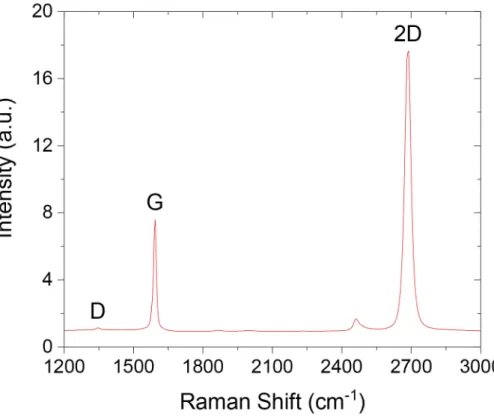Figure 3.14 Raman spectroscopy data obtained on the transferred graphene. D, G and 2D peaks are indicated 

### Measurement Results

In the C-V measurement, the AC signal voltage and frequency were set to 50 mV and 1 MHz. Then, the corresponding average flatband voltages are listed in Table 3.4 derived from Equations 2.58 and 2.59. As shown in Table 3.4, a large increase in the flat bandgap voltage is observed in the Al/graphene/SiO2/Si junction which is above 1 eV.

And the overall trend is quite similar to the result observed in the metal/graphene/GaAs junction. However, the drop in flat band voltage for Pt electrode is smaller than the Schottky barrier drop observed in the Pt/graphene/GaAs junction.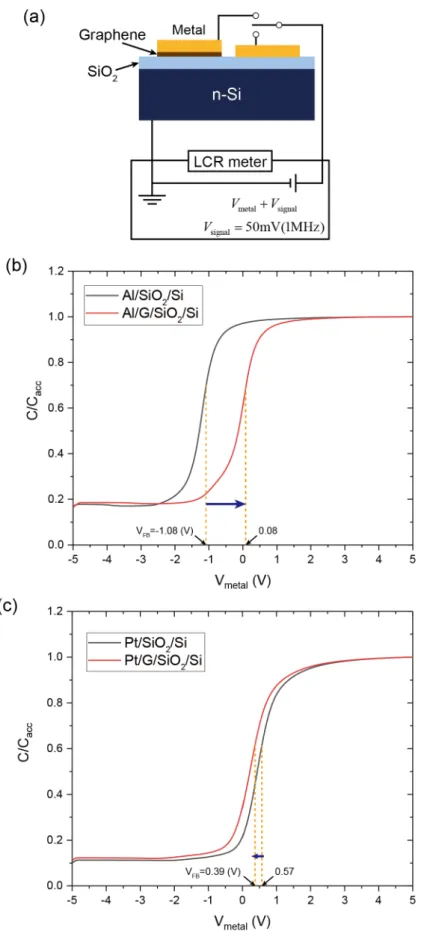Figure 3.15 (a) Schematic illustration of C-V measurement configuration and normalized capacitance curves for (a) Al and (b) Pt metal electrodes

### Analytic Calculation of Electrostatic Band Profile

Thus, by substituting equation 3.8 and 3.9 into equation 3.10, the fixed oxide charge 𝑄𝑄𝑜𝑜𝑖𝑖 can be calculated from the measured 𝑉𝑉𝐹𝐹𝐵𝐵. Finally, the interacting dipole charge density can be obtained from the charge neutrality condition as described in equation 3.18. As a result, Al and Al/graphene electrodes can be used as gate electrodes for CMOS logic circuits.

For example, it is possible to design a low-dimensional channel such as a nanowire electron channel with a tunable width or periodic quantum dots using the idea that the gate geometry can be modulated by a selectively inserted layer of graphene. For greater application, many low-dimensional quantum phenomena can be observed in a low-temperature environment.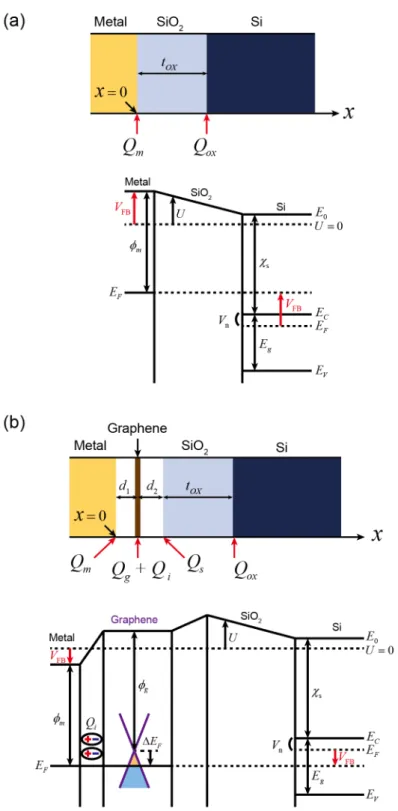Figure 3.16 Surface charge distribution and band diagram of (a) MOS and (b) MGOS junctions 

## Low-Temperature Application for Complementary MOSFET (CMOS) using Al Single Metal

### Experimental Analysis for Junctionless MOSFET with Silicide Contacts

50 nm thick Ni is deposited on the substrate using DC sputtering, followed by rapid thermal annealing process to form NiSi at 450 °C for 30 seconds in N2 atmosphere. The 30 nm thick Al2O3 gate oxide layer is deposited on the substrate using thermal atomic layer deposition (ALD). During the development process, Al is also etched into the developer, preventing direct contact of the photoresist with the graphene.

Finally, a 100 nm thick Al electrode is deposited using a thermal vaporizer, then the source, drain, and gate electrodes are isolated by photolithography, followed by a photoresist removal process. Consequently, using the threshold voltage shift induced by the intercalated graphene layer, we examine a MOSFET based on an intrinsic Si substrate with Ni silicide source and drain contacts to see the ambipolar current characteristics.

## Low-Dimensional Electron Channel Formation with Selectively Patterned Graphene

### Electrostatically-Controlled Nano-Ribbon Channel MOSFET

A schematic illustration of a nanostrip MOSFET channel and the boundary conditions used in the calculation are shown in Figure 4.9. Consequently, the conduction band profile of Si at the Si/SiO2 interface is calculated with a narrow electron channel as shown in Figure 4.10. The calculated parameters are summarized in Figure 4.11, and the exact values ​​are listed in Table 4.2 and Table 4.3.

Based on the CFMS, we construct the additional pump-out, helium supply line, and electrical measurement system as shown in Figure A.1. As shown in Figure B.1, two electrons in periodically oriented lattice can be paired by phonon-assisted interaction at low temperature for specific materials.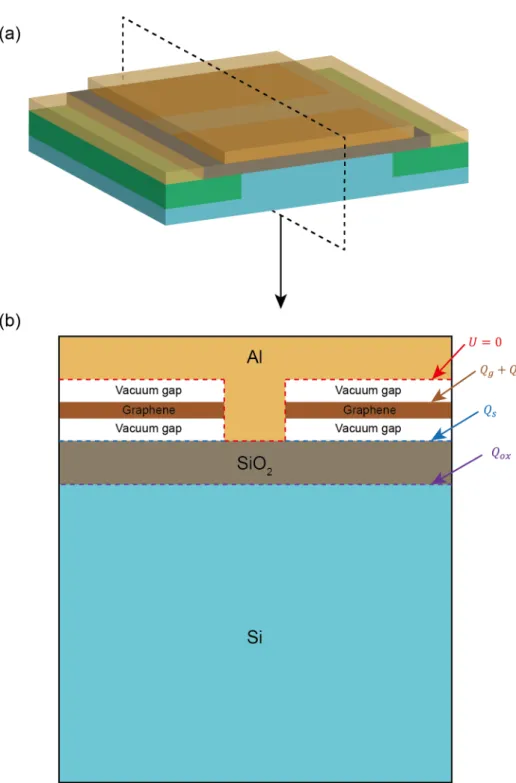Figure 4.9 (a) Schematic illustration of nano-ribbon channel intrinsic MOSFET controlled by selectively patterned graphene interlayer and (b) the boundary condition used in the calculation

Sample Fabrication

## Measurement Results and Discussion

Hu, Dual-function metal gate CMOS technology using metal interdiffusion, IEEE Electron Device Lett. Frey, Effective work function tuning in high κ metal oxide-semiconductor dielectric stacks by fluorine and lanthanide doping, Appl. Frohlich, Accurate determination of the effective work function of metal and solid oxide charge in MOS capacitors with high κ dielectric, Mater.

Ramprasad, Effective work function of metals coupled to dielectrics: a first-principles study of the Pt-HfO2 interface, Phys. Tianchun, An efficient nMOSCAP work function tuning method with high-k/metal gates with TiN/TaN double layer thickness, J.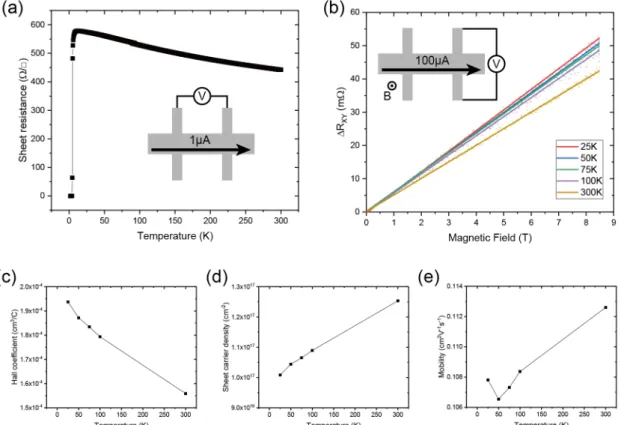Figure B.4. (a) Measured R-T curve and (b) Hall resistance obtained in the different temperatures with their Hall measurement configuration

수치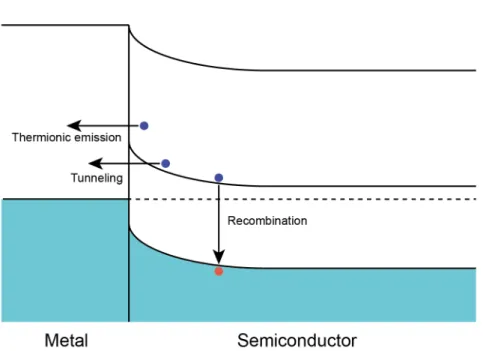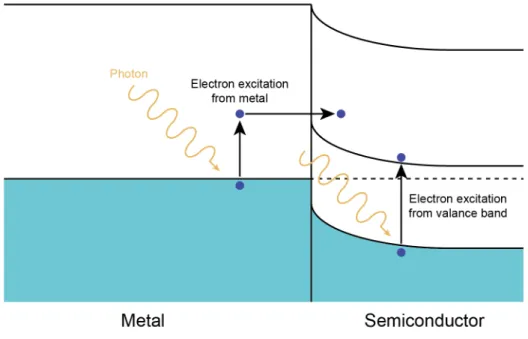+7

참조

관련 문서

Following Andrew et al. 1), resilience can be examined at the organizational level, which is defined as “the ability of organizations to work together

URE THE DEVICE IS CONSTRUCTED WITHIN TWO TUBS 4HE PLOYSILICON GATE EXTENDS OVER THE THIN GATE OXIDE AND TERMINATES ON THE THICK `ELD OXIDE 4HE E_ECT OF THE POLYSILI CON GATE

① The metal and semiconductor are assumed to be in intimate contact on an atomic scale, with no layers of any type (such as an oxide) between the components. ② There

Let’s establish analytical relationship for the charge density (ρ), the electric field ( E), and the electrostatic potential in an ideal MOS capacitor.. Because the metal

The uniform flow distribution of the primary coolant is required at the entrance region of the reactor core in SMART design, In three MCP(Main Coolant Pump) operation

Improvement of the Performance and Stability of Oxide Semiconductor Thin-Film Transistors Using Double-Stacked Active Layers.. Metal oxide semiconductor

By applying a sufficiently large positive gate voltage, we have inverted the surface of the semiconductor from a p-type to an n-type semiconductor.... MOS Capacitor :

In the first year of the cull, NE defined the maximum reduction level at 95% of the initial starting population (as opposed to the 70% minimum number) to avoid local extinction

• The Call Report forms for December 2017 are available on the Federal Financial Institutions Examination Council’s (FFIEC) website at

언어 선택하기

웹사이트는 선택한 언어로 번역됩니다.

기타 언어